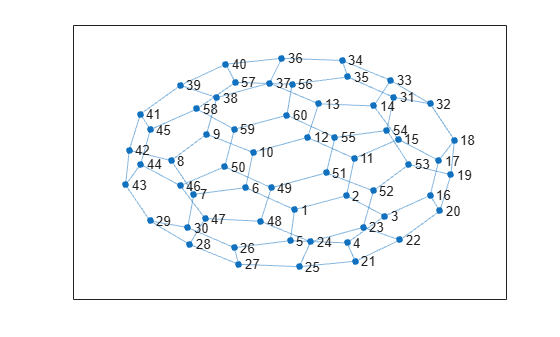# neighbors

Neighbors of graph node

## Syntax

``N = neighbors(G,nodeID)``

## Description

example

````N = neighbors(G,nodeID)` returns the node IDs of all nodes connected by an edge to the node specified by `nodeID`.```

## Examples

collapse all

Create and plot a graph, and then determine the neighbors of node `10`.

```G = graph(bucky); plot(G)````N = neighbors(G,10)`
```N = 3×1 6 9 12 ```

## Input Arguments

collapse all

Input graph, specified as a `graph` object. Use `graph` to create an undirected graph object.

Example: `G = graph(1,2)`

Node identifier, specified as one of the values in this table.

ValueExample
Scalar node index`1`
Character vector node name`'A'`
String scalar node name`"A"`

Example: `N = neighbors(G,3)`

Example: `N = neighbors(G,'A')`

## Output Arguments

collapse all

Neighboring nodes, returned as node indices if `nodeID` is numeric, or as node names if `nodeID` is a node name. A node that is connected to itself by an edge (a self-loop) is listed as its own neighbor only once.

## Version History

Introduced in R2015b

expand all

Behavior changed in R2018a